Heptagon 48　　-2012.08.28Up

I designed new puzzle and name it "Heptagon 48".
This puzzle is based on the 48 heptagons.

Will a regular heptagon tile a plane? The answer is NO.
But, it is possible if allowing for pentagonal space.

A “tetrahept” is a figure made of 4 regular heptagons joined edge to edge.
There are seven kinds of figures. 1)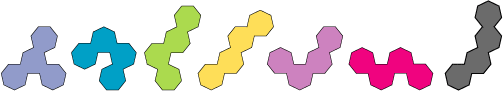This puzzle uses the following 6 kinds of tetrahepts.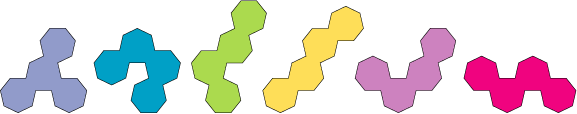[Goal]
The purpose of this puzzle is to fit the 2 sets of 6 tetrahepts (total 48 heptagons) into the space below.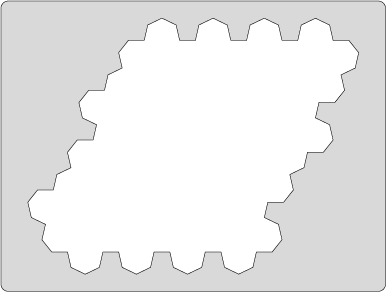[Mechanical Version]
Acctually, I have made the mechanical version of this puzzle out of two type of materials.
The first one is made out of wood. The other one is made out of marble, and this was submited to the Puzzle Design Competition 2012.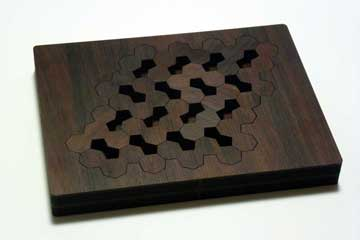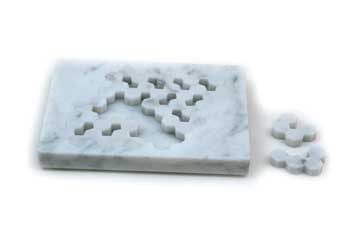Wood version of Heptagon 48 The competion version (marble)
[Further Information]
As mentioned above, regular heptagon can tile the plane if allowing for the pentagonal space. There are two types of this tiling, shown in Fig.1. Puzzle “Heptagon 48” uses the type 1 tiling in Fig 1. In this tiling, Six tetrahepts are compatible, although only five tetrahepts are compatible in the type 2.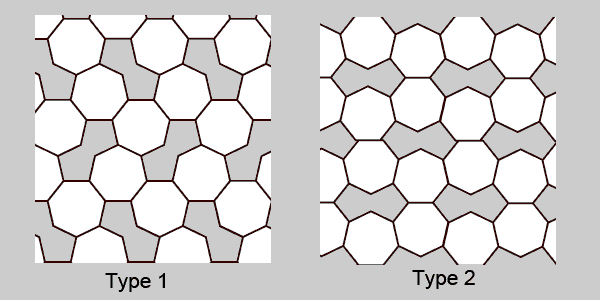Fig. 1

As for the basic of tiling, the following John J. G. Savard's site is very good 2). Here is the Basic Tilings Page.
But, we cannot see the same heptagonal tiling in this site and also others.

I found this two type of tiling in the process of my making idea for this puzzle. Surprisingly, however, this type of tiling occur in nature. It exists in the Boron-Chemistry and Carbon Nano-tube Chemistry. This figure is called "Pentaheptite". In the paper of "Pentaheptite Modifications of the Graphite Sheet" (J Chem Inf Comput Sci. 2000 Nov-Dec;40(6):1325-32.), it is described that pentaheptites (three-coordinate tilings of the plane by pentagons and heptagons only) are classified under the chemically motivated restriction that all pentagons occur in isolated pairs and all heptagons have three heptagonal neighbors 3). The example of pentaheptite nets in YCrB4 and ThMoB4 are also shown, and That is the same in Figure 1.

My puzzle was based on the findings that there can be heptagonal tiling with pentagonal space and six tetrahepts is compatible in the pentaheptite network (this heptagonal tiling). The number of solutions are not known yet, but I found 57 solutions manually until now. I think these solutions are rich in diversity than originally expected.

[Solution]
There are lots of solutions. Two examples of solutions are shown below.
I have found 57 solutions. The number of solutions of this puzzle is not known yet.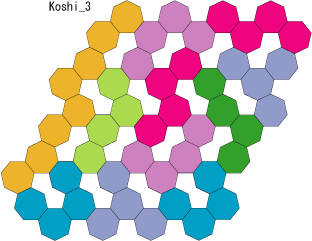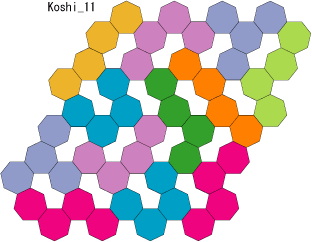lf you'd like to see the other solutions I have found, crick here.

--Koshi Arai (2012.09.06)

[Refference]

1. Tetrahepts:
George Sicherman's Site:
Polyform Curiosities   http://recmath.org/PolyCur/polycur.html
Catalogue of Polyhepts  http://recmath.org/PolyCur/polyhepts/index.html
Galvagni Figures for Polyhepts   http://recmath.org/PolyCur/hgal/index.html
Baiocchi Figures for Polyhepts   http://recmath.org/PolyCur/hbaiocchi/index.html

2. Heptagonal Tiling:
John J. G. Savard's Site: